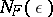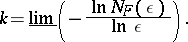# Metric dimension

A numerical characteristic of a compact set, defined in terms of coverings of "standard measure" , the number of which defines the metric dimension. Letbe a compact set, and letbe the minimal number of sets with diameter not exceedingthat are needed in order to cover. This function, depending on the metric in, takes integer values for all, and increases without bound as; it is called the volume function of. The metric order of the compact setis the numberThis quantity is not yet a topological invariant. Thus, the metric order of a curve in the sense of Jordan (cf. Line (curve)) with the Euclidean metric is equal to 1, but for a curve in the sense of Jordan passing through a perfect totally-disconnected set inof positive measure, this value is equal to. However, the greatest lower bound of the metric orders for all metrics on(called the metric dimension) is equal to the Lebesgue dimension (the Pontryagin–Shnirel'man theorem, 1931, see ).